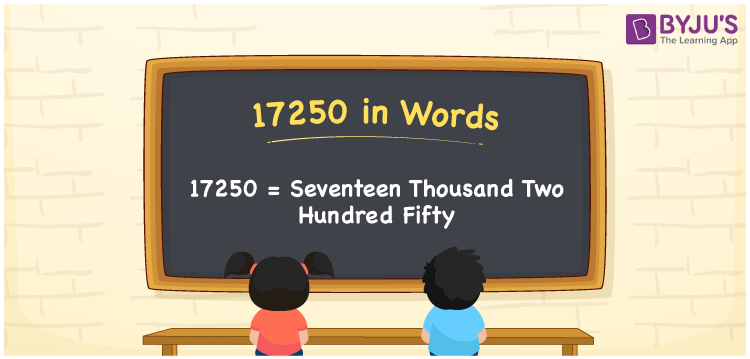# 17250 in Words

17250 in words is Seventeen Thousand Two Hundred Fifty. For example, if you have 17250 rupees in your account, then you can write, “I have Seventeen Thousand Two Hundred Fifty rupees in my account”. We know that the number name of any number can be written using the ones, tens, hundreds and thousands place of a number. Therefore, the place value chart is essential to write 17250 in words. 17250 is a cardinal number as it shows a specific quantity.

 17250 in words Seventeen Thousand Two Hundred Fifty Seventeen Thousand Two Hundred Fifty in Numbers 17250

## 17250 in English Words## How to Write 17250 in Words?

We can convert 17250 to words using a place value chart. This can be done as follows. The number 17250 has 5 digits, so let’s make a chart that shows the place value up to 5 digits.

 Ten thousands Thousands Hundreds Tens Ones 1 7 2 5 0

Thus, we can write the expanded form as:

1 × Ten thousand + 7 × Thousand + 2 × Hundred + 5 × Ten + 0 × One

= 1 × 10000 + 7 × 1000 + 2 × 100 + 5 × 10 + 0 × 1

= 17250

= Seventeen Thousand Two Hundred Fifty

17250 is the natural number that is succeeded by 17249 and preceded by 17251.

17250 in words – Seventeen Thousand Two Hundred Fifty

Is 17250 an odd number? – No.

Is 17250 an even number? – Yes

Is 17250 a perfect square number? – No

Is 17250 a perfect cube number? – No

Is 17250 a prime number? – No

Is 17250 a composite number? – Yes

## Frequently Asked Questions on 17250 in Words

### How to write 17250 in words?

17250 in English is written as “Seventeen Thousand Two Hundred Fifty”.

### Is the number 17250 an odd number?

No, the number 17250 is not an odd number.

### Is 17250 a composite number?

Yes, 17250 is a composite number.